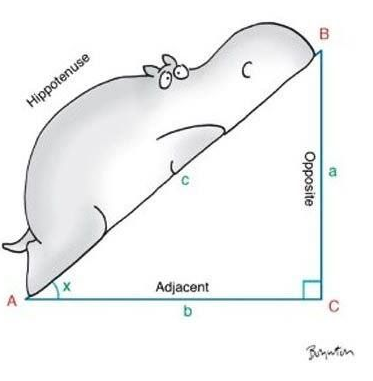Trigonometric Ratios TEST
3 years ago
mathgal
Save
Edit
Host a game
Live GameLive
Homework
Solo Practice
Practice25 QuestionsShow answers
• Question 1
300 secondsQ. A hawk sitting on top a tree branch spots a mouse on the ground 15 feet from the base of the tree.  The hawk swoops down toward the mouse at an angle of 30 degrees. What is the distance from the top of tree branch to the mouse?
7.5 ft
15 ft
26 ft
30 ft
• Question 2
180 secondsQ. Solve for COS A. SIMPLIFY THE FRACTION!
A
B
C
D
• Question 3
180 secondsQ. Solve for TAN A
A
B
C
D
• Question 4
180 secondsQ. Solve for SIN A. SIMPLIFY THE FRACTION!
A
B
C
D
• Question 5
300 secondsQ. Find the length of side w.
17.9 cm
18.0 cm
43.5 cm
43.6 cm
• Question 6
300 secondsQ. Solve for x. Round to the nearest tenth.
103.5
4.7
4.6
103.6
• Question 7
300 secondsQ. Find the measure of the indicated angle (?) round to the nearest WHOLE DEGREE!
39
55
34
56
• Question 8
120 secondsQ. Find the MEASURE of the MISSING ANGLE (in the upper right corner) of the right triangle shown.
90
50
40
CANNOT BE DETERMINED
• Question 9
60 seconds
Q. Which TRIG FUNCTION, does NOT use the HYPOTENUSE in its ratio?
sine
cosine
tangent
secant
• Question 10
300 secondsQ. Find the ratio for COS A. SIMPLIFY THE FRACTION!
36/27
4/5
36/45
3/5
• Question 11
300 secondsQ. A yacht is anchored 90 feet offshore from the base of a lighthouse. The angle of elevation from the boat to the top of the lighthouse is 26 degrees.The distance between the yacht and the top of the lighthouse is about 100 feet. Round answer to the nearest WHOLE NUMBER.
44 feet
39 feet
185 feet
205 feet
• Question 12
300 secondsQ. A tourist views a deer from a height of 45 feet.  The horizontal distance between the tourist and the deer is 130 feet.  At what angle (x) should the tourist hold his camera to photograph the deer? Round your answer to the nearest degree.
19 degrees
70 degrees
71 degrees
20 degrees
• Question 13
120 seconds
Q. To use the Pythagorean Theorem, we need...
A right triangle with 2 known angles  & 1 side
A right triangle with 2 known angles
A right triangle with 1 known side and 1 known angle
A right triangle with 2 known sides
• Question 14
30 seconds
Q. A triangle's 3 angles add up to...
90 Degrees
180 Degrees
360 Degrees
45 Degrees
• Question 15
120 seconds
Q. To use the TRIGONOMETRY, we need...
A right triangle with 2 known angles  & 1 side
A right triangle with 2 known angles
A right triangle with 1 known side and 1 known angle
A right triangle with 2 known sides
• Question 16
120 secondsQ. What would you use to solve for "a"?
sin
cos
tan
Pythagorean theorem
• Question 17
120 seconds
Q. How do you find the "inverse of sin" on your calculator?
sin, sin-1
sin-1, sin-1
2nd key, sin1
2nd key, sin-1
• Question 18
30 seconds
Q. What type of triangle is the context of our study of trigonometry & Pythagorean Theorem?
right triangle
isosceles triangle
scalene triangle
acute triangle
• Question 19
180 secondsQ. Find Cos(C) as a decimal rounded to the nearest ten-thousandth.
.4706
1.8750
.8824
.5333
• Question 20
180 secondsQ. Find Tan(A) as a decimal rounded to the nearest ten-thousandth.
.4706
1.875
.8824
.5333
• Question 21
180 secondsQ. Find the length of side y. ROUND ANSWER TO THE NEAREST TENTH!
69.8 cm
21.3 cm
76.3 cm
70.0 cm
• Question 22
180 secondsQ. Solve for x. ROUND ANSWER TO THE NEAREST TENTH!
29.7
29.8
4.9
5.0
• Question 23
120 secondsQ. Choose the correct ratio.
sin-1(12/29)
sin-1(29/12)
tan-1(12/29)
tan-1(29/12)
• Question 24
300 secondsQ. Find the MEASURE OF ANGLE "X" to the nearest WHOLE DEGREE!
22
67
68
23
• Question 25
120 seconds
Q. When do you use the "INVERSE TRIG FUNCTION" on your CALCULATOR?